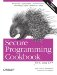# Determining a Days Number Within a Given Year

### Determining a Day s Number Within a Given Year

Problem

You want to determine a day's number within a given year. For example, January 1 is the first day of each year; February 5 is the 36th day of each year, and so on. But since some years have leap days, after February 28, a given day doesn't necessarily have the same numbering each year.

Solution

The solution to this problem requires the solution to several problems simultaneously. First, you have to know how many days are in each month, which, in turn, means you have to know how to determine whether a year is a leap year. Example 5-9 provides routines for performing these computations.

Example 5-9. Routines for determining a day's number within a given year

```#include

using namespace std;

enum MonthEnum {
jan = 0, feb = 1, mar = 2, apr = 3, may = 4, jun = 5,
jul = 6, aug = 7, sep = 8, oct = 9, nov = 10, dec = 11
};

bool isLeapYear(int y) {
return (y % 4 == 0) && ((y % 100 != 0) || (y % 400 == 0));
}

const int arrayDaysInMonth[] = {
31, 28, 31, 30, 31, 30, 31, 31, 30, 31, 30, 31
};

int n;
int arrayFirstOfMonth[] = {
n = 0,
n += arrayDaysInMonth[jan],
n += arrayDaysInMonth[feb],
n += arrayDaysInMonth[mar],
n += arrayDaysInMonth[apr],
n += arrayDaysInMonth[may],
n += arrayDaysInMonth[jun],
n += arrayDaysInMonth[jul],
n += arrayDaysInMonth[aug],
n += arrayDaysInMonth[sep],
n += arrayDaysInMonth[::oct],
n += arrayDaysInMonth[nov]
};

int daysInMonth(MonthEnum month, int year) {
if (month == feb) {
return isLeapYear(year) ? 29 : 28;
}
else {
return arrayDaysInMonth[month];
}
}

int firstOfMonth(MonthEnum month, int year) {
return arrayFirstOfMonth[month] + isLeapYear(year);
}

int dayOfYear(MonthEnum month, int monthDay, int year) {
return firstOfMonth(month, year) + monthDay - 1;
}

int main( ) {
cout << "July 1, 1971, was the " << dayOfYear(jul, 1, 1971);
cout << " day of the year" << endl;
}```

The program in Example 5-9 outputs the following:

`July 1, 1971, was the 181 day of the year`

Discussion

The code in Example 5-9 is a relatively straightforward but useful set of functions for working with dates and leap years. Notice that I have abandoned what I call the "document and pray" approach used in the previous recipes. What I mean by this is that the months are no longer represented by indexes but rather enumerations. This significantly reduces the chance of programmer error when passing a month to a function as an argument.

The leap year computation shown in Example 5-9 is in accordance to the modern Gregorian calendar. Every fourth year is a leap year, except every hundredth year unless that year is divisible by 400 (e.g., 1896 was a leap year, 1900 wasn't, 2000 was, 2004 was, 2100 will not be).Secure Programming Cookbook for C and C++: Recipes for Cryptography, Authentication, Input Validation & More
ISBN: 0596003943
EAN: 2147483647
Year: 2006
Pages: 241